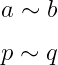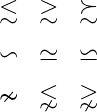# How to denote similar-to(∼) symbol in LaTeX?

Similar-to symbol is a logical-mathematical symbol denoted by ∼. Latex stores more than one command and package that represents a similar-to symbol.

Symbol Similar to
Type Logical
Package Default
Command \sim
Example \sim
\documentclass{article}
\begin{document}
$a \sim b$
$p \sim q$
\end{document}

Output :The best practice is to use the direct \sim command without installing any packages.

And multiple logical symbols are used with the similar-to symbol. Such as equal, gatearthan, lessthen etc.

\documentclass{article}
\usepackage{amssymb,amsmath}
\begin{document}
$\begin{matrix} \lesssim & \gtrsim & \succsim\\[6pt] \backsim & \simeq & \backsimeq\\[6pt] \nsim & \lnsim & \gnsim \end{matrix}$
\end{document}

Output :#### Md Jidan Mondal

LaTeX expert with over 10 years of experience in document preparation and typesetting. Specializes in creating professional documents, reports, and presentations using LaTeX.